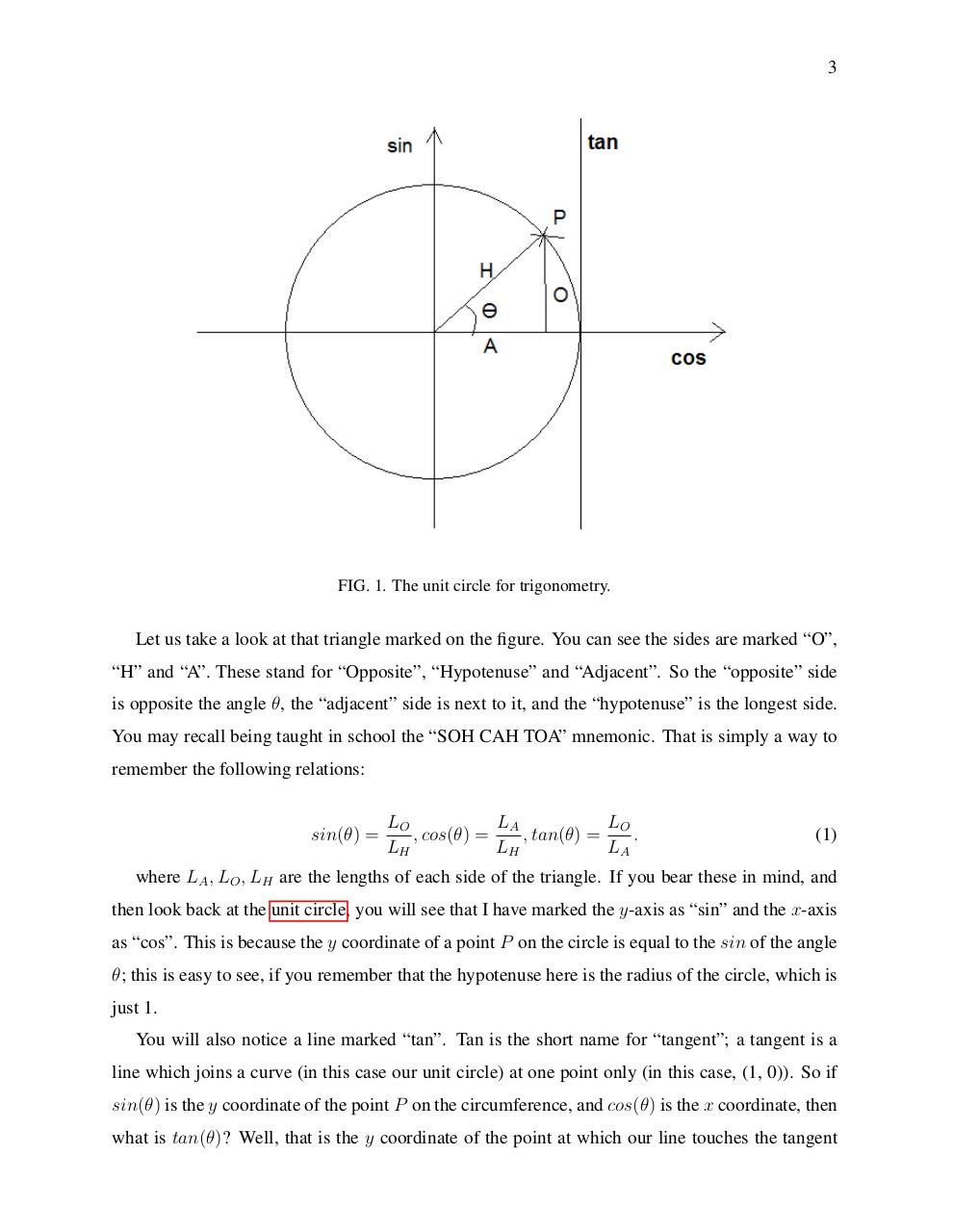# GDMathsGuide.pdfPage 1 2 34515

#### Text preview

3

FIG. 1. The unit circle for trigonometry.

Let us take a look at that triangle marked on the figure. You can see the sides are marked “O”,
“H” and “A”. These stand for “Opposite”, “Hypotenuse” and “Adjacent”. So the “opposite” side
is opposite the angle θ, the “adjacent” side is next to it, and the “hypotenuse” is the longest side.
You may recall being taught in school the “SOH CAH TOA” mnemonic. That is simply a way to
remember the following relations:

sin(θ) =

LO
LA
LO
, cos(θ) =
, tan(θ) =
.
LH
LH
LA

(1)

where LA , LO , LH are the lengths of each side of the triangle. If you bear these in mind, and
then look back at the unit circle, you will see that I have marked the y-axis as “sin” and the x-axis
as “cos”. This is because the y coordinate of a point P on the circle is equal to the sin of the angle
θ; this is easy to see, if you remember that the hypotenuse here is the radius of the circle, which is
just 1.
You will also notice a line marked “tan”. Tan is the short name for “tangent”; a tangent is a
line which joins a curve (in this case our unit circle) at one point only (in this case, (1, 0)). So if
sin(θ) is the y coordinate of the point P on the circumference, and cos(θ) is the x coordinate, then
what is tan(θ)? Well, that is the y coordinate of the point at which our line touches the tangent